## 1.1 Introduction

Since video can be viewed as a sequence of pictures, video coding can be seen as an extension to image compression. Compression is the process of compacting data, reducing the number of bits. With eye specific features and removal of redundant data we can achieve compression. Compression involves a complementary pair of systems, a compressor (enCOder) and a decompressor (DECoder) and hence the name CODEC, the system performs that performs encoding & decoding.

## 1.2 Human Visual system

Rods and cones senses the light focused by the lens on retina. Rods provide sensation on the intensity of light and cone the sensation on color. There are three types of cones each corresponds to red, green and blue. Figure 1.1 shows the sensitivity of eye towards various wavelengths.

##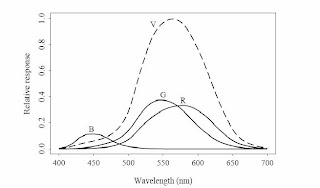Figure 1.1 Response of eye towards different wavelength

Curve R, G & B shows the sensitivity of cones towards wave length. The curve V shows the response of eye intensity variation. The value of V can be represented as V (λ) α 2R + G + B/20, where R, G and B is the intensity of Red, Green and Blue wavelengths. From the Figure 1.1 it’s clear that Eye is more sensitive towards light intensity variation than color variation. This is the principle behind color sub-sampling.

`

## 1.1 Picture Representation in Digital Domain

This section describes how an image is represented, provides an idea about pixels, resolution and RAW format.

### 1.1.1 Pixel

As you all know we can derive any color by properly combining the primary colors, Red, Green and Blue. Figure 1.2 shows the formation of secondary colors from the primary colors. In the case of analog picture representation we have the values of Red, Green and Blue intensities or a combination of these intensities ie: the effective color, at each and every point on the space. But in Digital domain we divide the space (the image focused by the lens) into a finite number of square patterns. This finest square area is called pixel, derived from the word picture element. A Pixel gives the value of Red, Green and Blue intensities in that area.

The intensity values of Red, Green and Blue components are separated by some filter arrangement called Baye’s Filter. This finite division of space is performed by image sensors: CCD (Charge coupled devices), CID (Charge injection Devices), Active pixel CMOS.

Figure 1.3 illustrates the Spatial sampling of a grey scale image into digital domain. The value at each position is approximated to any of 256 levels. Then we need 8 bits/pixel for representing the intensity value.In the case of color image we need 8 bits for representing each of the primary color, which results in 24 bits/pixel. And we have 224 = 16,777,216 color levels

for each pixel. Number of bits/pixel is called color depth.

Figure 1.2 Formation of 20

Colors from the 10 colors

##Figure 1.3 Spatial sampling of analog scene

### 1.1.2 Resolution

The numbers of pixels used to represent an image can be said in terms of resolution. Higher the number of pixels higher will be the clarity. Resolution determines the maximum enlargement possible for an image. For getting good quality pictures there should be a minimum of 300pixels/inch. Figure 1.4 shows an image sampled at different resolution.

##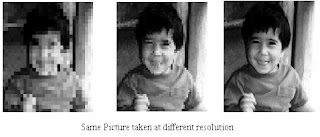Figure 1.4

### 1.1.3 RAW Formats

RAW Format implies that there is no compression done on the image. The major types of RAW format are RGB, YUV, YIQ. As explained in section 1.2, our eye is more sensitive towards light intensity variation than color variation. So loss on color information will not affect the over all quality of the image. RGB is an end stream format. Information from the image sensor is in RGB format and we need the same format for displaying the image on an end

device. YUV & YIQ formats are developed for Analog TV transmission (NTSC & PAL respectively) and the digital version of YUV, YCbCr is the most common format used for image and video compressions.

Conversion from one format to another is described below:

RGB to YCbCr Conversion

Y = 0.299 R + 0.587 G + 0.11B

Cb = 0.564 (B - Y)

Cr = 0.713 (R - Y)

YCbCr to RGB

R = Y + 1.402 Cr

G = Y – 0.344 Cb – 0.714 C r

B = Y + 1.722 Cb

Y – Luminance Signal

Cb, Cr – Chrominance Signal, Color difference signal

R – Red

G – Green

B – Blue

## 1.2 Need for Compression

Consider an image of resolution 640 × 480. Let us calculate the size of the picture in RAW format. Each of the 10 Color is represented by 8 bits. Then for each pixel it needs 24 bits. Total no of pixels in the image is 640 × 480 = 307200 pixels. Therefore the size of the image turns to 307200 × 3 bytes = 921600 bytes. But an image in compressed format with the same resolution takes only 100 KB.

In the case of RAW video stream of length 1 sec its needs 640 × 480 × 3 × 25 = 23040000 bytes (23 MB) of storage if the frame rate is 25 frames/sec. But it’s known that the VCD format video having a size 700 MB plays for around 80 minutes. In the former case we need 110400 MBs (23 MB × 60 × 80) as storage space for 80 minutes video. Therefore we can achieve a high compression 150: 1 at the cost of computational complexities.

## 1.3 Generic Method of Image Compression

The various steps involved in image compression using JPEG encoder is explained using the block diagram in Figure 1.5.

##Figure 1.5 Block Diagram of JPEG Encoder

### 1.3.1 Color Space Conversion

The image obtained as the output of image sensor will be in RAW format, RGB. Suppose RAW image has a resolution 640 × 480. First step is to change it into another color space YCbCr. Y corresponds to the intensity variation and Cb, Cr are called color difference signals.

### 1.3.2 Chroma Subsampling

Color space conversion utilizes the sensitivity of eye in luminous part of image. Retaining the luma (Y signal) and sub-sampling the Chroma ( Cb & Cr ) we represent the image in a reasonably good manner. The commonly used subsampling formats are 4:4:4, 4:2:2 and 4:2:0. The numbers indicate the relative sampling rate of each component in the horizontal direction. In 4:4:4 (Figure 1.8) sampling for every four luminance samples there are four Cb and four Cr samples. In 4:2:2 (Figure 1.7) sampling, the chrominance components have the same vertical resolution as the luma but half the horizontal resolution (the numbers 4:2:2 mean that for every four luminance samples in the horizontal direction there are two Cb and two Cr samples). 4:2:2 video is used for high-quality color reproduction. In the popular 4:2:0 (Figure 1.6) sampling format, each Cb and Cr have half the horizontal and vertical resolution of Y. JPEG image compression is based on 4:2:0 subsampling.

Figure 1.6Figure 1.7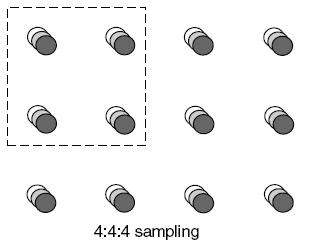Figure 1.8

Chroma Subsampling results in the reduced number of bits to represent the image.

Example:

Image resolution: 640 × 480 pixels

Y resolution: 640 × 480 samples, each represented with eight bits

4:4:4 :- Cb, Cr resolution: 640 × 480 samples, each eight bits

Total number of bits: 640 × 480 × 8 × 3 = 7372800 bits

4:2:0 :- Cb, Cr resolution: 320 × 240 samples, each eight bits

Total number of bits: (640 × 480× 8) + (320 × 240 × 8 × 2) = 3686400 bits

The 4:2:0 version requires half as many bits as the 4:4:4 version

4:2:2 :- Cb, Cr resolution: 320 × 480 samples, each eight bits

Total number of bits: (640 × 480× 8) + (320 × 480 × 8 × 2) = 4915200 bits.

The 4:2:2 version requires 2/3 as many bits as the 4:4:4 version.

### 1.3.3 MacroBlock

Statistical analysis reveals that images are spatially redundant (ie the pixels are found highly correlated to the neighboring ones) and removing the spatial redundancy itself results in high compression. Consider the block specified in Figure 1.9 which represents a homogenous region where the pixel values of block (Table 1.1) are found closely related. Studies revealed that the optimal size of a block is 8 × 8 for utilizing the spatial redundancy of an image and the 8 × 8 block is called a macroblock. Another advantage in using the macroblock size of 8 x 8 is low memory requirement. Macroblock can be viewed as square matrix of dimension 8.

Followed by chroma subsampling we divide the entire image into macro blocks. An image of resolution 640 × 480 can be divided into 4800 Y macroBlocks, 1200 Cb & 1200 Cr macroblocks in case of 4:2:0 subsampling.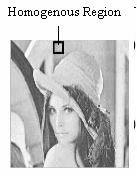Figure 1.9 Table 1.1 Pixel Values corresponding to MacroBlock shown in Figure 1.9

Next step is to take Discrete Cosine Transform for each of these macroblocks.

### 1.3.4 Transform Coding (Discrete Cosine Transform)

Discrete Cosine transform is a technique to represent the data spread over the macroblock into a lesser number of significant values. DCT (Discrete Cosine Transform)

represents any pattern (image) as the weighted sum of the basis patterns. On taking DCT, we will get a matrix of the same dimension as that of macroblock, which shows how much of each pattern is present in the image. Figure 1.10 shows the basis patterns for 8 x 8 block Each of these pattern is called frequency and the value obtained on DCT is the amplitude of that frequency in the macroblock.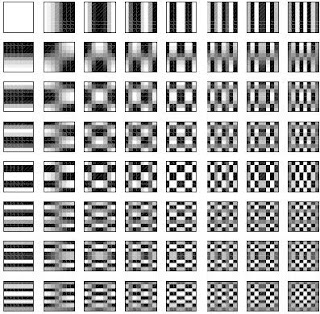Fig 1.10 8 × 8 DCT basis patternsFigure 1.11 Illustration of DCT

Figure 1.11 illustrates the concept of DCT. On taking DCT for the original pattern shown in Figure 1.11 we will get weights corresponding to positions (0,0), (0,2), (2,0) in the basis pattern. The patterns present in original macroblock are Average DC value, Vertical frequency & Horizontal frequency respectively. Similar is the case for any image.

#### 1.3.4.1 Mathematical Formulation of DCT

The Discrete Cosine Transform (DCT) operates on X, a block of N × N samples (typically image samples) and creates Y, an N × N block of coefficients. The action of the DCT (and its inverse, the IDCT) can be described in terms of a transform matrix A. The forward DCT (FDCT) of an N × N sample block is given by:

Y = AXAT eqn 1.1

And inverse DCT (IDCT)

X = ATYA eqn 1.2

where X is a matrix of samples(macroblock), Y is a matrix of DCT coefficients and A is an N × N transform matrix. The elements of A are

Ai j = Ci cosWhere Ci =(i =0), Ci =(i > 0) eqn 1.3

Yxy = Cx CyXijcoseqn 1.4

Xij =Cx Cy Yxycoseqn 1.5

The transform matrix A for 8 × 8 DCT is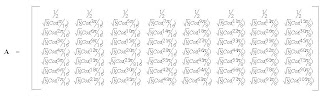Example :Calculating DCT for the macroblock defined in Figure 1.9X =

Table 1.2 Pixel values corresponding to macroblock shown in Figure 1.9Y =

Table 1.3 The transform matrix Y is obtained using the eqn 1.1

Value of Y (Table 1.3), shows that most of the energy gets crowded to low coefficients. Even approximating the amplitude of higher coefficient values to zero will not make much effect on the image. That is what we observed from the experiments. It’s achieved by the process called Quantization.

### 1.3.5 Quantization

Experiments had already proved that our eye has a very poor response towards high intensity variation. ie our eye will not notify the absence of patterns in the high frequency side (those patterns comes in the lower right side of Figure 1.10). Thus we can achieve better compression with loss of data. So it’s called lossy compression. Quantizer helps to attain this. A quantiser maps a signal with a range of values X to a quantised signal with a reduced range of values Y. It should be possible to represent the quantised signal with fewer bits than the original since the range of possible values is smaller. A scalar quantiser maps one sample of the input signal to one quantised output value and a vector quantiser maps a group of input samples (a ‘vector’) to a group of quantised values. Here we are explaining the scalar quantiser, one commonly used in image and video compression.

Zij = Round ( ) eqn 1.6

Where Z is output of quantiser, Y is the transform matrix, Q is the Quantization matrix. The rescaled value on inverse quantization is

Y’ij = Zij × Qij eqn 1.7

Where Y’ij is the value in the matrix obtained on inverse quantization. i & j are the indices of matrix.

Two different quantization matrices are used in the case of color image, One for the luma and the other for chroma.Table 1.4 Quantization matrix used for luminance signal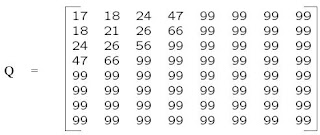Table 1.5 Quantization matrix used for chrominanace signal

Example:

Consider the macroblock defined in Figure 1.9. The output of quantizer for the macro block using eqn 1.6 comes as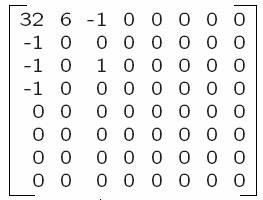Z =

Table 1.6 Quantized value corresponding to transform matrix, Y shown in Table 1.3

The rescaled matrix Y’ as per the eqn 1.7 is shown as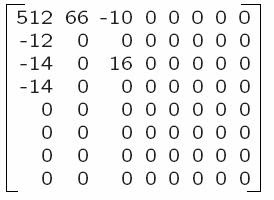Y’ =

Table 1.7 The rescaled Transform matrix from Z using eqn 1.7

Taking inverse transform, IDCT on Y’ we will get the reconstructed pixel value matrix X’ as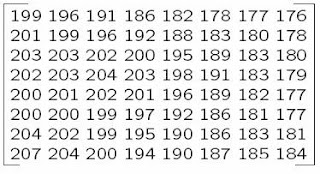X’ =

Table 1.8 Reconstructed pixel value matrix of the macroblock shown in figure 1.9

The difference between the original and reconstructed macroblock pixel values is shown as Δ matrix. Its very clear there is only a slight difference between the original macroblock pixel values and the reconstructed macroblock.

Δ = X – X’ eqn 1.8Δ =

Table 1.9 The error value from original pixel value

### 1.3.6 Zig Zag Scan

The quantizer ignores all the insignificant high frequency values as shown in Table1.6. The 8 x 8 matrix gives 64 values. Most of the high frequency values are approximated to zero. By efficiently representing the zeros we can achieve high compression. For this purpose Zig Zag scan and Run length encoding are introduced.

Figure 1.12 Zig Zag Scanning

Zig-zag scanning technique groups the non-zero and runs of zero and represent the data in a linear array . Run length encoding represents the runs zero in an efficient manner. The quantized values are reordered in a zig-zag manner to produce a linear array by taking values in the order of position (0,0), (0,1), (1,0), (2,0), (1,1), (1,2), (0,3)…. It’s explained in the Figure 1.12

By scanning in the Zig-Zag pattern, the values of Z matrix (Table 1.6) can be represented in a linear array as 32, 6, -1, -1, 0, -1, 0, 0, 0, -1, 0, 0, 1, 0, 0, 0, 0…

### 1.3.7 Run Level Coding.

It’s a method to represent the runs of zeros efficiently. The linear array obtained by zig-zag scanning is processed to produce a series of (zero-run, level) pairs. Zero-run will give the number of zeros preceding a non zero value. Level represents the signed magnitude of non-zero value.

General rule for run level coding:

First identify the non zero values. Count the number of zeros preceeding the non-zero as zero-runs, and the non-zero value as level. For example the Run level coding of the above linear array results in an array of pairs like (0, 32), (0, 6), (0, -1), (0, -1), (1,-1), (3, -1), (2, 1), (EOB). ‘EOB’ (End of Block) indicates that the remaining coefficients are zero.

### 1.3.8 Entropy encoding

Our aim is to represent the image with a less number of bits. So far we represent the values, like DCT coefficients or Run Length coded sequences with fixed number of bits. But statistical studies of image compression reveal that some values come frequently. Encoding those values, which contribute a major share of image, with less number of bits can leads to an efficient compression ratio Variable length coding (VLC) is used here. The various algorithms used for VLC are :

Shannon-FanoAlgorithm

Huffman coding

Arithmetic coding

Based on these algorithms some tables are prepared and indications to these tables are signaled in the header of JPEG bit stream format.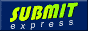Search Engine Optimization and SEO Tools

1.Great stuff. But some images are not visible.

2.can you use a jpeg decoder to decode Mpeg i frame or vice versa

3.hey thanks...good stuff

4.Cool stuff really useful, esp the DCT coefficient diagram

5.This comment has been removed by a blog administrator.

6.I also found similar kind of blog at wordpress except it has pros & cons of various video codecs

http://videocodecs.wordpress.com/

7.In principle, a good happen, support the views of the author

8.Thanks for this nice structured topic,very useful

9.good stuff on fundamentals...

http://vidtechtrends.blogspot.com

10.Mohammad Javad SalehiMarch 25, 2011 at 6:44 AM

thanks a lot,very very nice stuff

11.Thank you for the info. It sounds pretty user friendly. I guess I’ll pick one up for fun. thank u
Freevi

12.Kumar Sharma EkaveeraAugust 9, 2011 at 9:23 PM

Really excellent information on fundas :)

13.interesting! although I have no expert, but I want have to know more and more, on your blog just interesting and useful information.

14.Great website, looks very clean and organized. Keep up the good work!

15.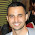In main profile B slice reference lists description:
"in increasing picture order count"

Should be "in decreasing picture order count" , at least according to the example.

16.Thank you for sharing such a useful information.

regards,
melbourne web designer

17.Awesome information. Get much help from here. I am using this Image compression free

18.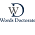I read this article. I think You put a lot of effort to create this article. I appreciate your work.
thesis Writing Service

19.Thank you for sharing such a informative information with us. Keep on sharing the blog like this.
International Business Services & Support Platform
merger and acquisition service
joint venture services